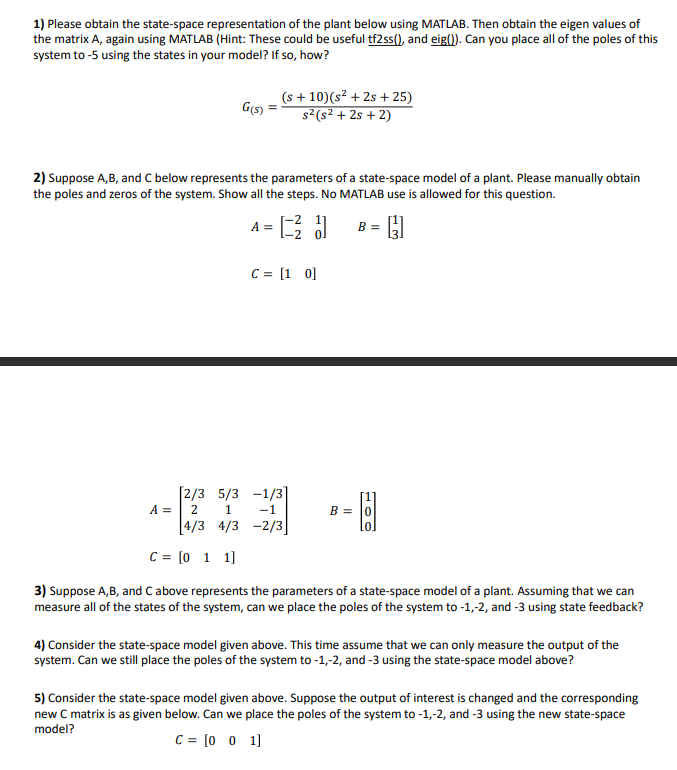# (Solved): 1) Please obtain the state-space representation of the plant below using MATL ...???????

1) Please obtain the state-space representation of the plant below using MATLAB. Then obtain the eigen values of the matrix A, again using MATLAB (Hint: These could be useful tf2ss(), and eig()). Can you place all of the poles of this system to $$-5$$ using the states in your model? If so, how? $G_{(s)}=\frac{(s+10)\left(s^{2}+2 s+25\right)}{s^{2}\left(s^{2}+2 s+2\right)}$ 2) Suppose $$A, B$$, and $$C$$ below represents the parameters of a state-space model of a plant. Please manually obtain the poles and zeros of the system. Show all the steps. No MATLAB use is allowed for this question. \begin{aligned} A & =\left[\begin{array}{ll} -2 & 1 \\ -2 & 0 \end{array}\right] \quad B=\left[\begin{array}{l} 1 \\ 3 \end{array}\right] \\ C & =\left[\begin{array}{ll} 1 & 0 \end{array}\right] \end{aligned} $\begin{array}{ll} A & =\left[\begin{array}{ccc} 2 / 3 & 5 / 3 & -1 / 3 \\ 2 & 1 & -1 \\ 4 / 3 & 4 / 3 & -2 / 3 \end{array}\right] \\ C & =\left[\begin{array}{lll} 0 & 1 & 1 \end{array}\right] \end{array}$ 3) Suppose $$A, B$$, and $$C$$ above represents the parameters of a state-space model of a plant. Assuming that we can measure all of the states of the system, can we place the poles of the system to $$-1,-2$$, and $$-3$$ using state feedback? 4) Consider the state-space model given above. This time assume that we can only measure the output of the system. Can we still place the poles of the system to $$-1,-2$$, and $$-3$$ using the state-space model above? 5) Consider the state-space model given above. Suppose the output of interest is changed and the corresponding new $$\mathrm{C}$$ matrix is as given below. Can we place the poles of the system to $$-1,-2$$, and $$-3$$ using the new state-space model? $C=\left[\begin{array}{lll} 0 & 0 & 1 \end{array}\right]$

We have an Answer from Expert